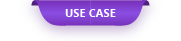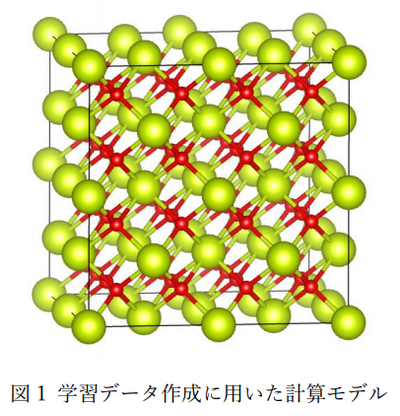The neural network molecular dynamics method (NNMD) enables faster simulations than first-principles calculations and more accurate simulations than existing molecular

# Behavior analysis of solid-liquid interface of CeO2 using neural network molecular dynamics method

The neural network molecular dynamics method (NNMD) enables faster simulations than first-principles calculations and more accurate simulations than existing molecular dynamics calculations, but the first-principles for creating interatomic potentials for use in NNMD. The challenge is the need for large amounts of computationally-based teacher data. In this case, the potential learning is performed in a short time by simultaneously executing a large amount of first-principles calculations at once using a cloud system to create learning data, and CeO 2 is difficult to reproduce in the conventional force field . We will introduce an example of analyzing the behavior of the solid-liquid interface using NNMD.

### 1. Creation of training data for NNMD potential

Figure 1 shows the computational model used to create the training data for CeO 2 . In addition, Table 1 shows the details of the calculation conditions. Deep MD-kit  was used for potential machine learning.

In this case, in order to create training data, first-principles molecular dynamics calculations that impose a wide range of temperature and pressure conditions were executed many times. However, by using the cloud system, we were able to execute all first-principles calculation degrees at the same time and perform potential learning in a short time (Fig. 2, Table 2). This time, 80 cases of first-principles molecular dynamics calculation are executed for the calculation of 80,000 training data.

### 2. Benchmark calculation

The Deep MD-kit used in this case is compatible with GPU and can learn potential at high speed. In addition, the created potential parameters can be used with the free molecular dynamics calculation code LAMMPS, and MD calculation also supports MPI parallel and GPU acceleration. Table 3 shows the results of comparing the calculation time when training 80,000 training data in Table 1 between CPU and GPU. In potential learning, it can be seen that the GPU can be about 3 to 4 times faster than the CPU.

Table 4 shows the results of speed comparison between CPU and GPU for MD calculation using the model shown in Fig. 3. In MD calculation, it was confirmed that GPU is about 10 times faster than CPU.

### 3. Simulation of solid-liquid interface of CeO 2

The results of simulating the solid-liquid interface of CeO 2 using the created potential function are shown below. MD calculation of 500ps was performed at 1atm and 2000K using a calculation model of 1500 atoms (total number of atoms: 3000 atoms) in both solid phase and liquid phase. It was confirmed that the liquid phase gradually changed from the interface to the solid phase. As a result, it can be expected that NNMD can be used to calculate phenomena that are difficult to carry out due to the high calculation cost of first-principles calculations and the absence of force field parameters.

### 4. References

 Han Wang, Linfeng Zhang, Jiequn Han, and Weinan E. “DeePMD-kit: A deep learning package for many-body potential energy representation and molecular dynamics.” Computer Physics Communications 228 (2018): 178-184.

Original Source from: https://ctc-mi-solution.com/ニューラルネットワーク分子動力学法を用いたceo2/